# Rutherford Island Width Evolution Equation

Equations (11.149) and (13.1) yield the generalized Rutherford equation for a rotating magnetic island chain interacting with a static resonant magnetic perturbation (see Section 12.4):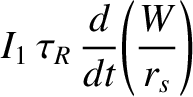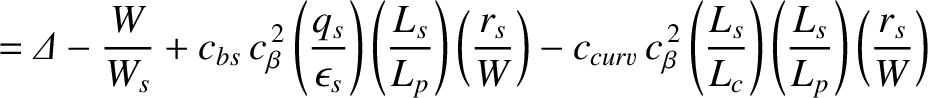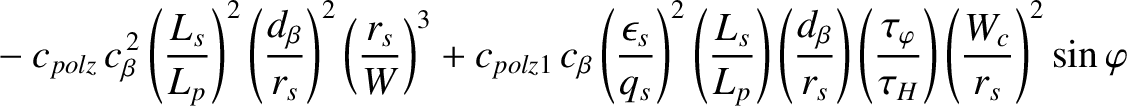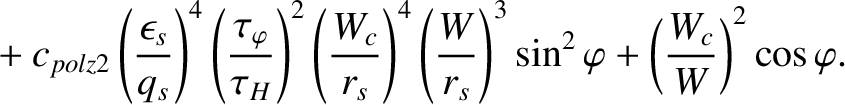(13.4)

Here,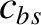,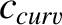, and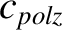are defined in Equations (12.16), (12.17), and (12.18), respectively, whereas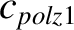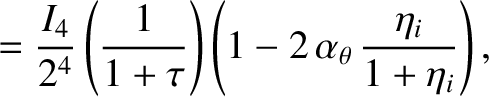(13.5)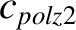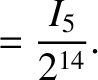(13.6)

Moreover,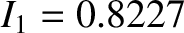,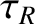is the resistive diffusion time [see Equation (5.49)],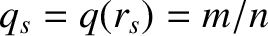the safety-factor at the rational surface [see Equation (3.2)],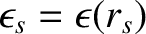the inverse aspect-ratio at the rational surface [see Equation (3.18)],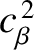a dimensionless measure of the plasma pressure at the rational surface [see Equations (4.65) and (4.66)],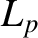the effective pressure gradient scale-length at the rational surface [see Equation (8.35)],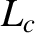the magnetic curvature length at the rational surface [see Equation (11.57)],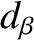the ion sound radius [see Equation (4.75)],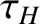the hydromagnetic time [see Equation (5.43)],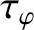the toroidal momentum confinement time [see Equation (5.50)],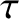the ratio of the electron and ion pressure gradients at the rational surface [see Equation (4.5)], and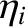a dimensionless measure of the ion temperature gradient at the rational surface [see Equation (4.3)]. Finally, the dimensionless ion neoclassical poloidal flow-damping parameter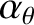is defined in Equation (12.6), and the dimensionless integrals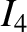and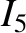are specified in Figure 11.1. Note that, in writing Equation (13.4), we have assumed that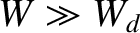[see Equation (12.20)] (i.e., that the island chain is sufficiently wide that the plasma pressure profile is flattened within its magnetic separatrix).

The first term on the right-hand side of Equation (13.4) is the linear tearing stability index,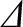. The second term is a stabilizing (i.e., negative) saturation term . The third term is a destabilizing (i.e., positive) term that is due to the loss of the bootstrap current within the magnetic separatrix of the island chain consequent on the flattening of the plasma pressure profile in this region . The fourth term is a stabilizing term due to magnetic field-line curvature [13,18]. The fifth term represents the stabilizing effect of the ion polarization current induced in the vicinity of the rational surface when the ion fluid is diverted around the island chain's magnetic separatrix [7,25,26,27]. The sixth and seventh terms are additional ion polarization terms that arise from the modified ion flow in the immediate vicinity of the island chain when the chain is subject to an externally generated electromagnetic torque [10,27]. The final term is due to the direct interaction between the island chain and the resonant magnetic perturbation .

It is convenient to rewrite Equation (13.4) in the form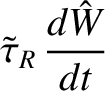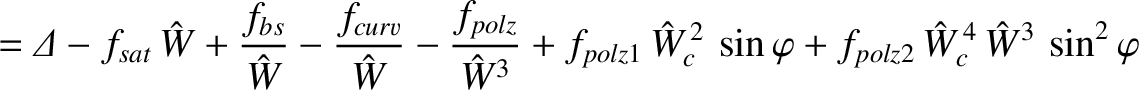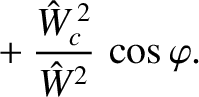(13.7)

Here,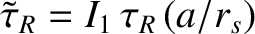,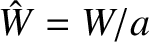, and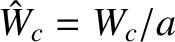, where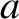is the plasma minor radius. Moreover,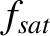,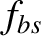,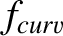, and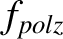are defined in Equations (12.23)–(12.26), respectively, whereas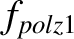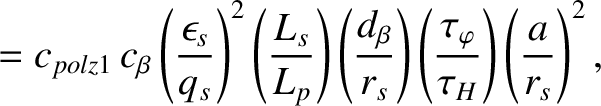(13.8)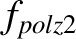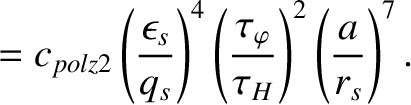(13.9)

Table 13.1: Dimensionless parameters characterizing the generalized Rutherford equation in a low-field and a high-field tokamak fusion reactor.
 Low-Field High-Field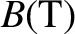5.0 12.07.47 7.470.712 0.712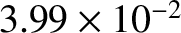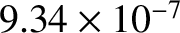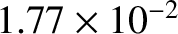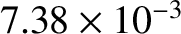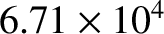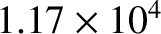Table 12.1 gives estimates for the various dimensionless parameters that characterize the right-hand side of the generalized Rutherford equation, (13.7), for the case of a low-field and a high-field tokamak fusion reactor. (See Chapter 1.) These estimates are made using the following assumptions: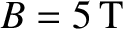(low-field) or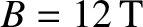(high-field),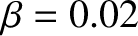,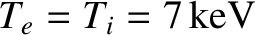,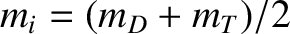(where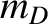and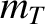are the deuteron and triton masses, respectively),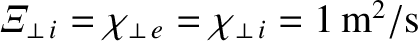,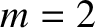,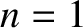,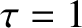,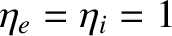, and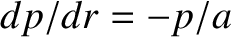. The plasma equilibrium is assumed to be of the Wesson type with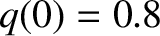,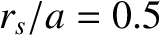, and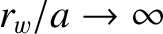. (See Section 9.4.)